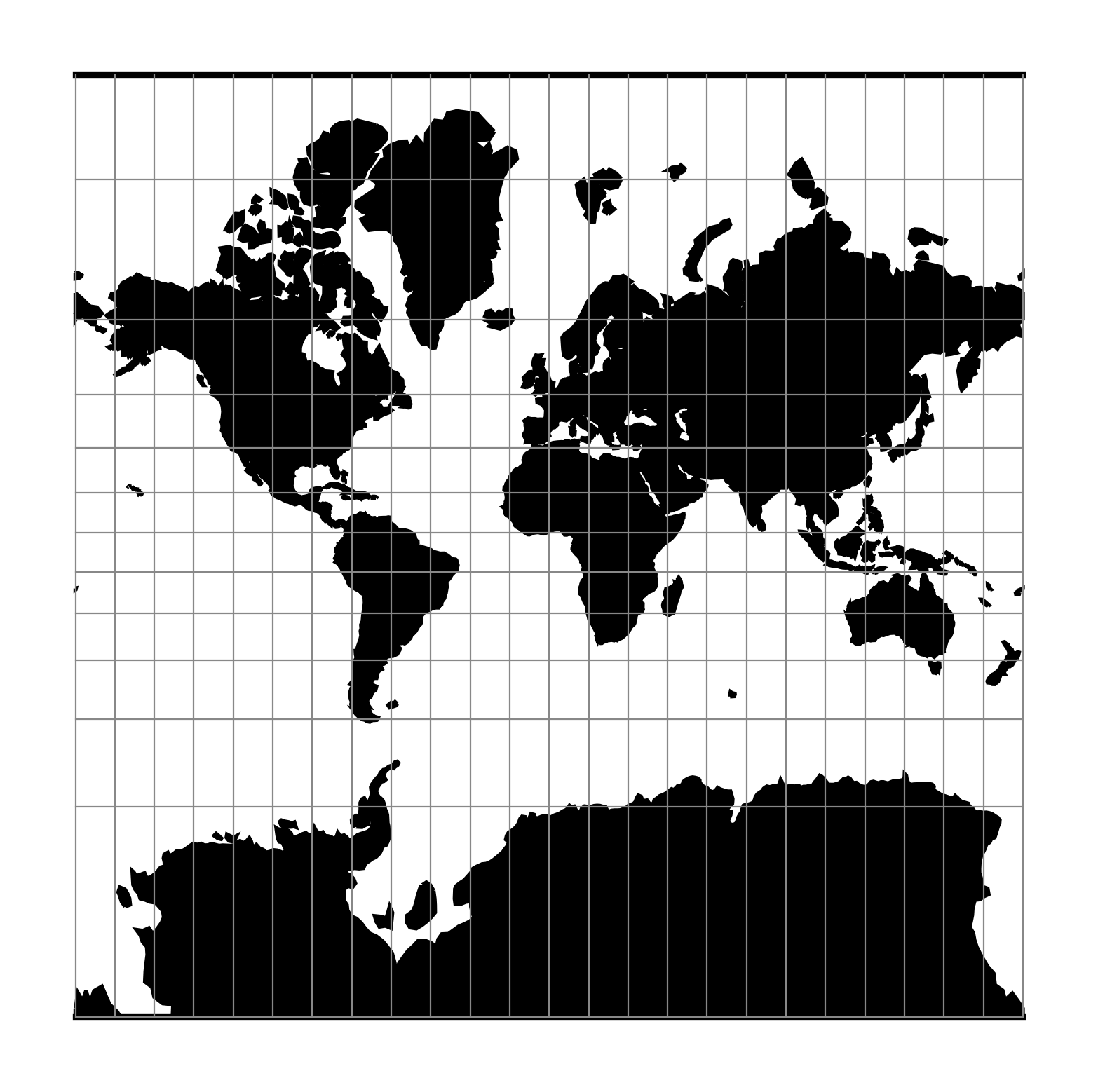# Mercator¶

The Mercator projection is a cylindrical map projection that origins from the 15th century. It is widely recognized as the first regularly used map projection. The projection is conformal which makes it suitable for navigational purposes.

 Classification Conformal cylindrical Available forms Forward and inverse, spherical and elliptical projection Defined area Global, but best used near the equator Alias merc Domain 2D Input type Geodetic coordinates Output type Projected coordinatesproj-string: +proj=merc

## Usage¶

Applications should be limited to equatorial regions, but is frequently used for navigational charts with latitude of true scale (+lat_ts) specified within or near chart’s boundaries. Often inappropriately used for world maps since the regions near the poles cannot be shown [Evenden1995].

Example using latitude of true scale:

\$ echo 56.35 12.32 | proj +proj=merc +lat_ts=56.5
3470306.37    759599.90


Example using scaling factor:

echo 56.35 12.32 | proj +proj=merc +k_0=2
12545706.61     2746073.80


Note that +lat_ts and +k_0 are mutually exclusive. If used together, +lat_ts takes precedence over +k_0.

## Parameters¶

Note

All parameters for the projection are optional.

+lat_ts=<value>

Latitude of true scale. Defines the latitude where scale is not distorted. Takes precedence over +k_0 if both options are used together.

Defaults to 0.0.

+k_0=<value>

Scale factor. Determines scale factor used in the projection.

Defaults to 1.0.

+lon_0=<value>

Longitude of projection center.

Defaults to 0.0.

+x_0=<value>

False easting.

Defaults to 0.0.

+y_0=<value>

False northing.

Defaults to 0.0.

+ellps=<value>

See proj -le for a list of available ellipsoids.

Defaults to “GRS80”.

+R=<value>

Radius of the sphere given in meters. If used in conjunction with +ellps +R takes precedence.

## Mathematical definition¶

The formulas describing the Mercator projection are all taken from G. Evenden’s libproj manuals [Evenden2005].

### Spherical form¶

For the spherical form of the projection we introduce the scaling factor:

$k_0 = \cos \phi_{ts}$

#### Forward projection¶

$x = k_0 \lambda$
$y = k_0 \ln \left[ \tan \left(\frac{\pi}{4} + \frac{\phi}{2} \right) \right]$

#### Inverse projection¶

$\lambda = \frac{x}{k_0}$
$\phi = \frac{\pi}{2} - 2 \arctan \left[ e^{-y/k_0} \right]$

### Elliptical form¶

For the elliptical form of the projection we introduce the scaling factor:

$k_0 = m\left( \phi_{ts} \right)$

where $$m\left(\phi\right)$$ is the parallel radius at latitude $$\phi$$.

We also use the Isometric Latitude kernel function $$t()$$.

Note

m() and t() should be described properly on a separate page about the theory of projections on the ellipsoid.

#### Forward projection¶

$x = k_0 \lambda$
$y = k_0 \ln t \left( \phi \right)$

#### Inverse projection¶

$\lambda = \frac{x}{k_0}$
$\phi = t^{-1}\left[ e^{ -y/k_0 } \right]$